# Sequences

Sequences and series:

In this unit we will look at the power of sequences to describe patterns.

Syllabus points: 1.2, 1.3, 1.4

Sequence and series are a good starting point to begin the syllabus as they are quite accessible – and the shared content across the different courses allows students t change courses early on.

I have made 13 worksheets and activities to assist with sequences lessons.  They are:

1. A Sequences Puzzle [investigation]
2. Arithmetic sequences and series 1
3. Arithmetic sequences and series 2
4. Compound interest
5. Geometric sequence and series 1
6. Geometric sequence and series 2
7. Mixed exam style questions SL
8. Mixed exam style questions HL.
9. Some sequence puzzles [short]
10. Test:  Sequences
11. Treasure hunt: compound interest
12. Treasure hunt: arithmetic
13. Treasure hunt: geometric

You can scroll down below to see pictures of the questions.  You can download both the questions and mark scheme from the bottom of the page.

A sequences puzzle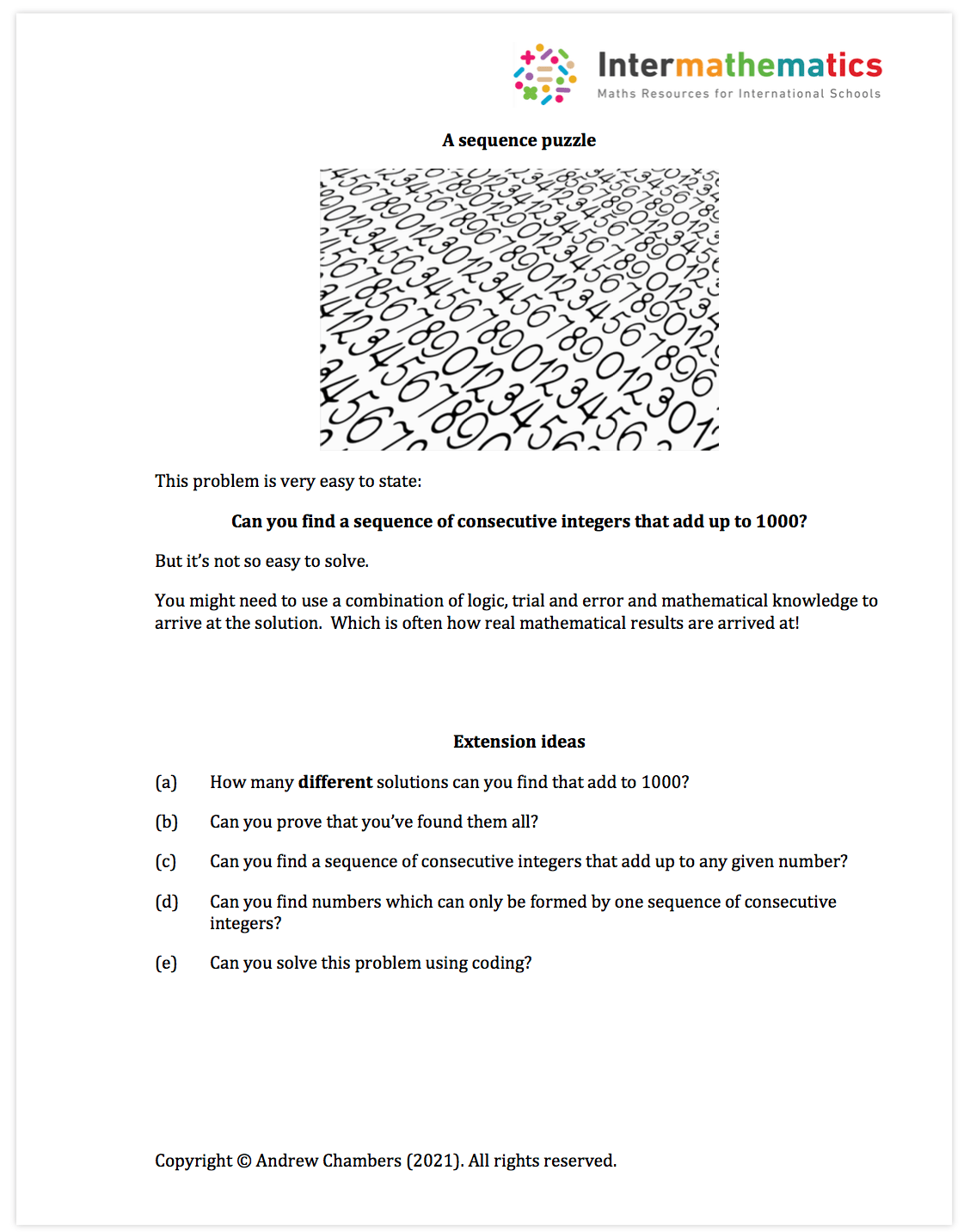Note:  This is an activity which lends itself to a classroom investigation or for homework.  This introduces the idea of how an exploration could begin.

Arithmetic sequences and series 1:Note:  This is a short worksheet which can be used in lesson or for homework.  It covers syllabus point: SL 1.2 with a focus on arithmetic sequences.

Arithmetic sequences and series 2:Note:  This is a short worksheet which can be used in lesson or for homework.  It covers syllabus point: SL 1.2 with a mixture of sequences and series.

Compound interest: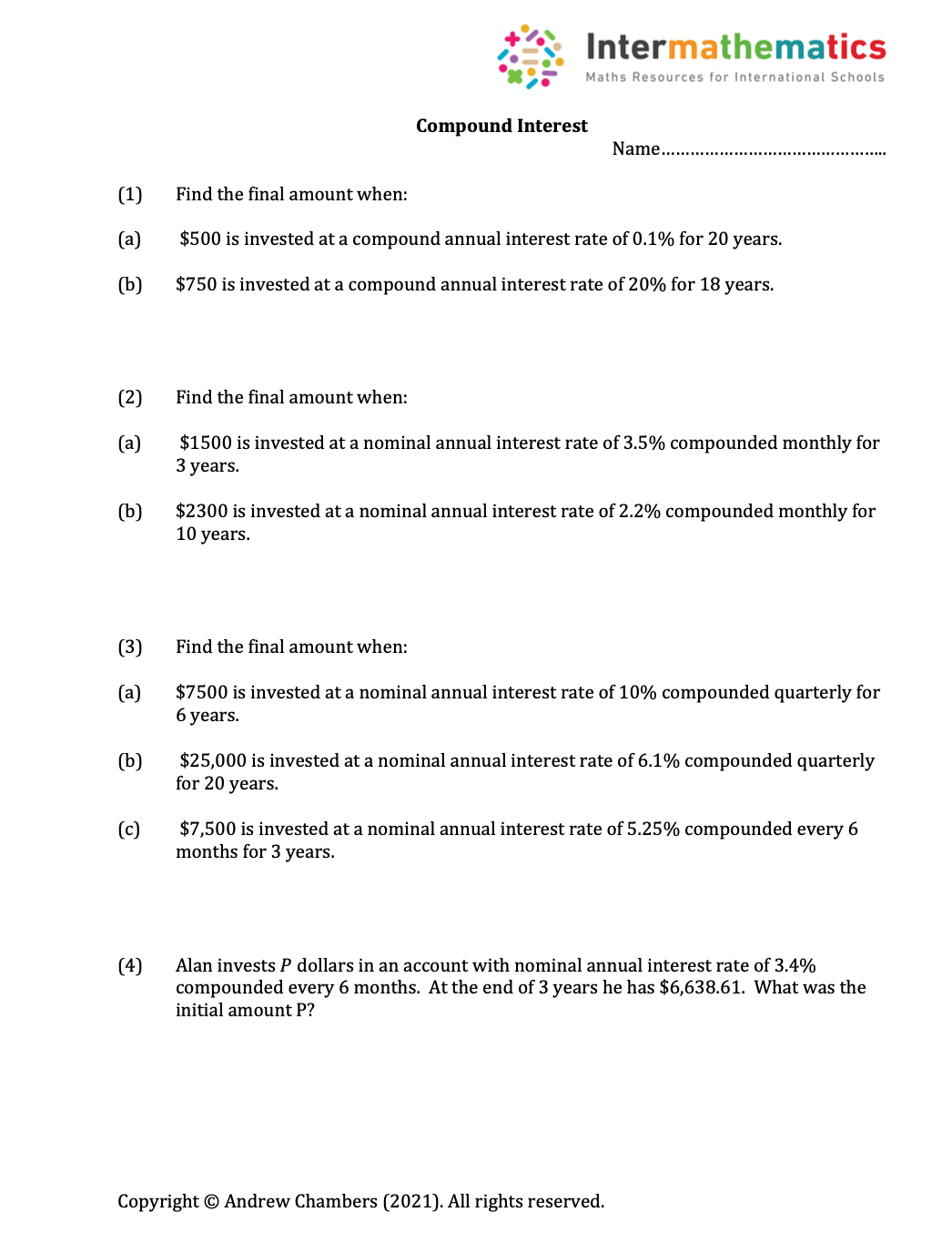Note:  This is a short worksheet which can be used in lesson or for homework.  It covers syllabus point: SL 1.4 with a mixture of compounding problems.

Geometric sequence and series 1:Note:  This is a short worksheet which can be used in lesson or for homework.  It covers syllabus point: SL 1.3 with a mixture of geometric sequences and series.

Geometric sequence and series 2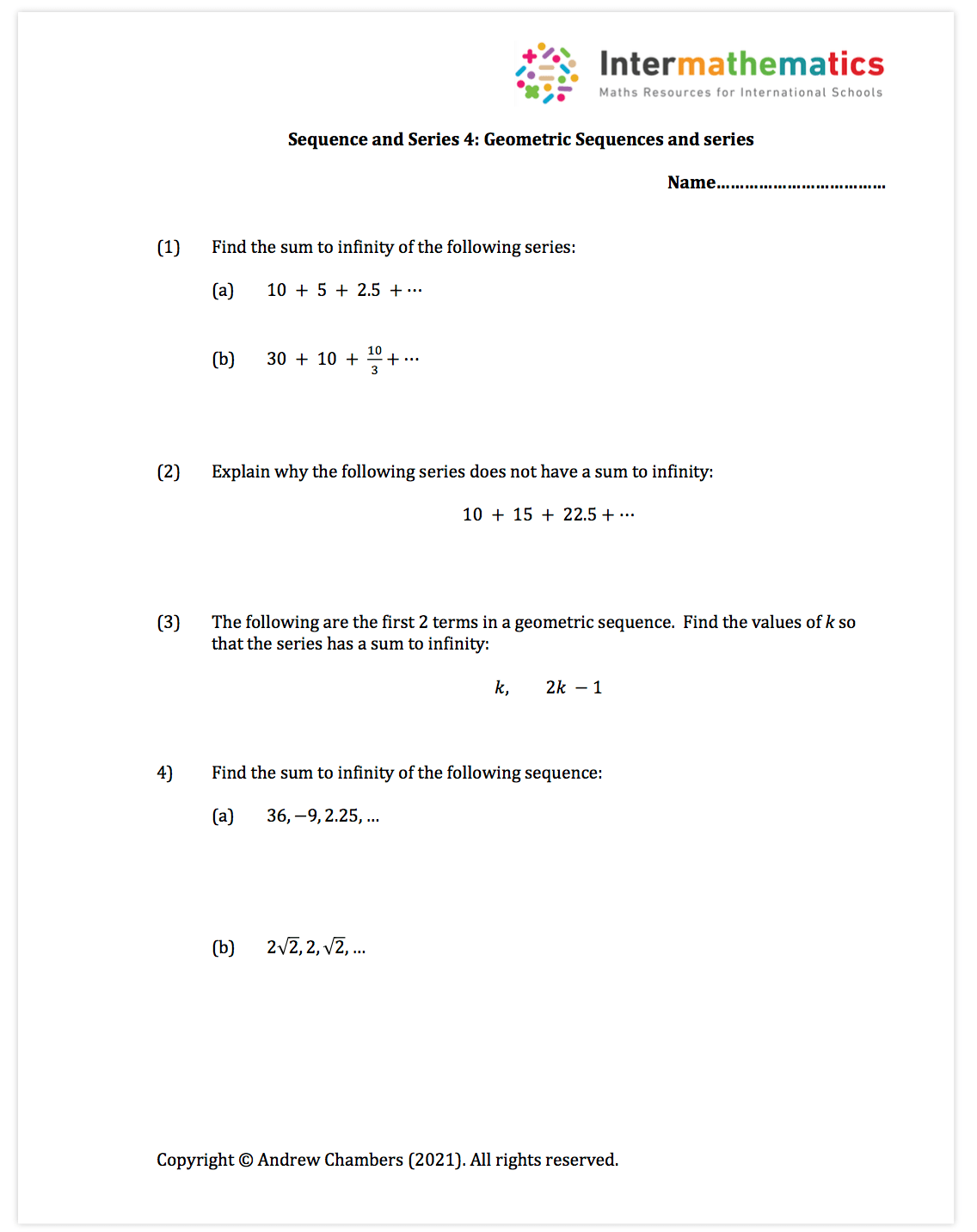Note:  This is a short worksheet which can be used in lesson or for homework.  It covers syllabus point: SL 1.3 and SL 1.8 with a mixture of geometric sequences and series including sums to infinity.

Mixed exam style questions SL: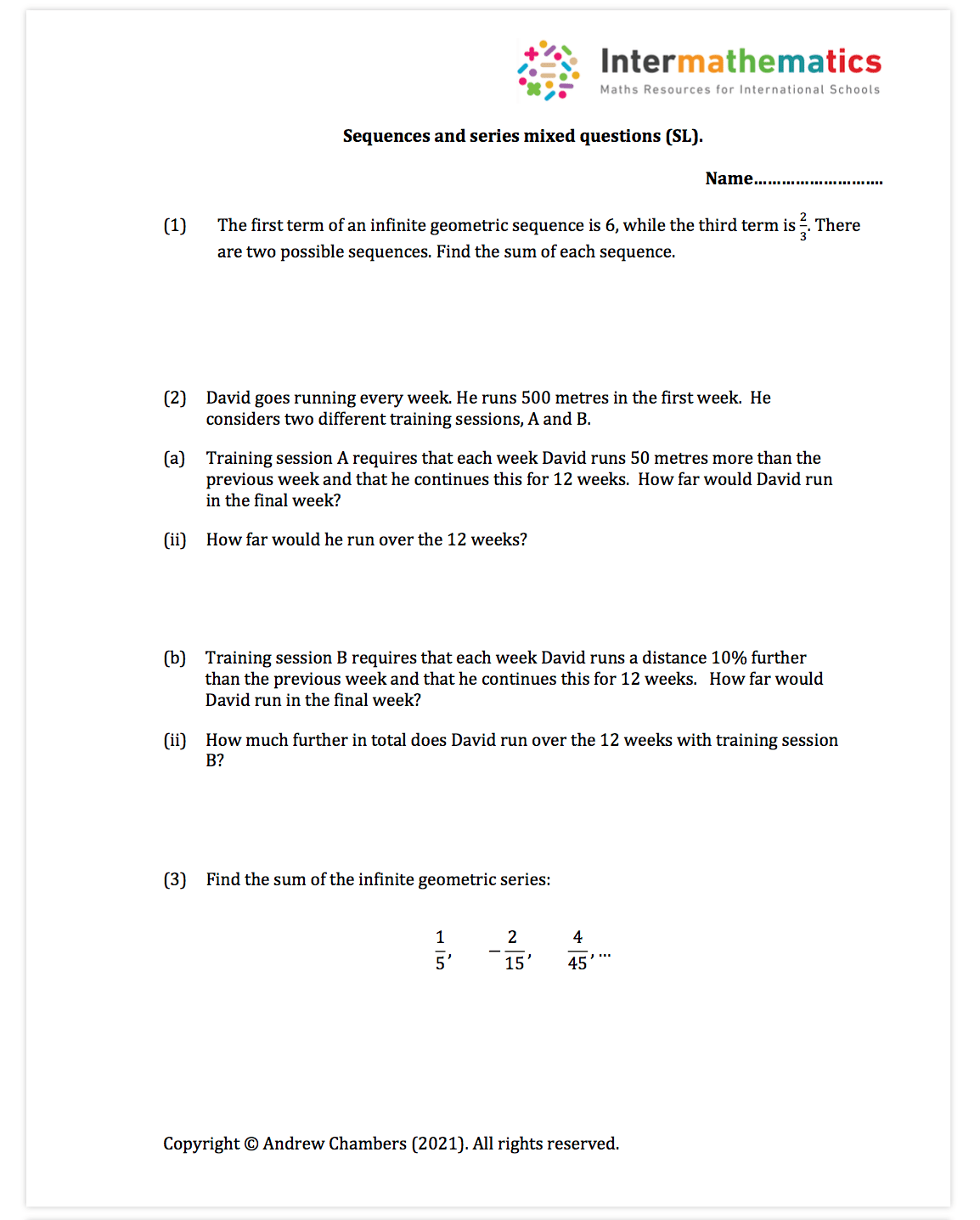Note:  This is a short worksheet which can be used in lesson or for homework and gives some past paper SL style questions  covering SL1.2-SL1.4 and also SL1.8.

Mixed exam style questions HL:Note:  This is a short worksheet which can be used in lesson or for homework and gives some HL past paper style questions  covering SL1.2-SL1.4 and also SL1.8.  These include more problem solving examples.

Some sequence puzzles [short]Note:  This can be used as a student worksheet, or as a teacher starter activity to get students investigating number puzzles.

Test:  Sequences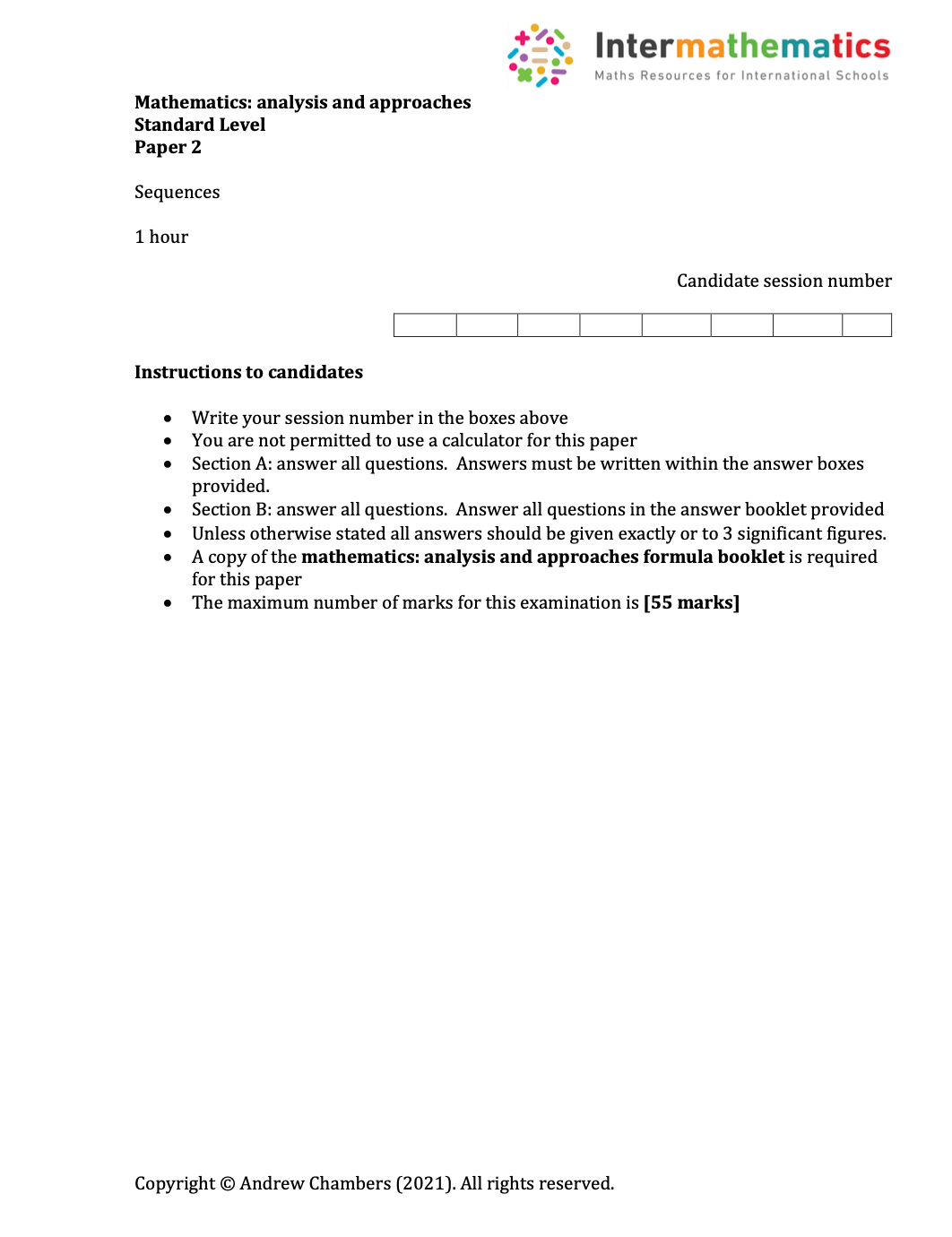Note:  This is a one hour test on the sequences topic – designed for SL students.

Treasure hunt: Compound interest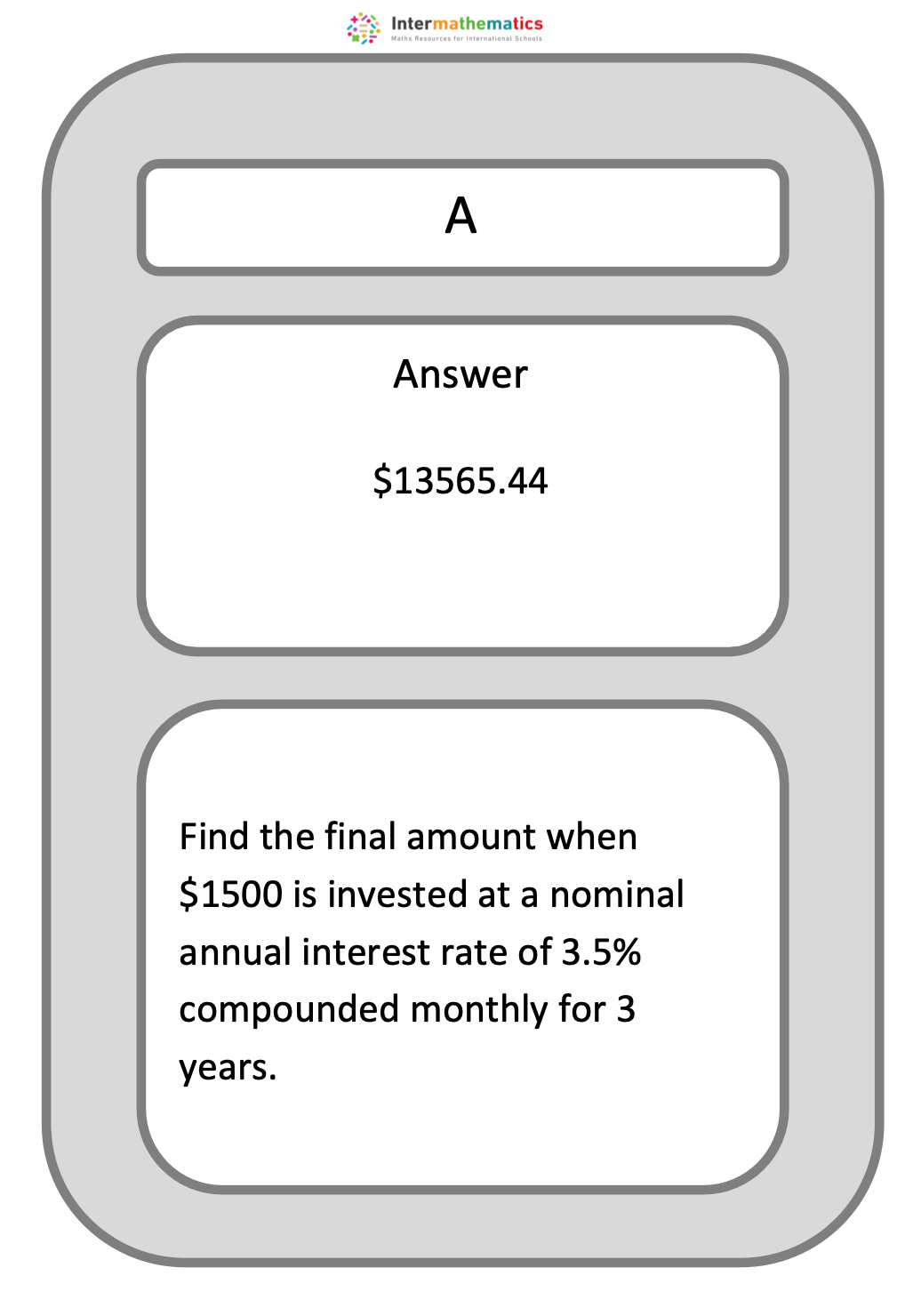Note:  This is an activity which can be done in pairs or as a whole class activity.  Students solve a mixture of compound interest problems.

Treasure Hunt:  Arithmetic sequences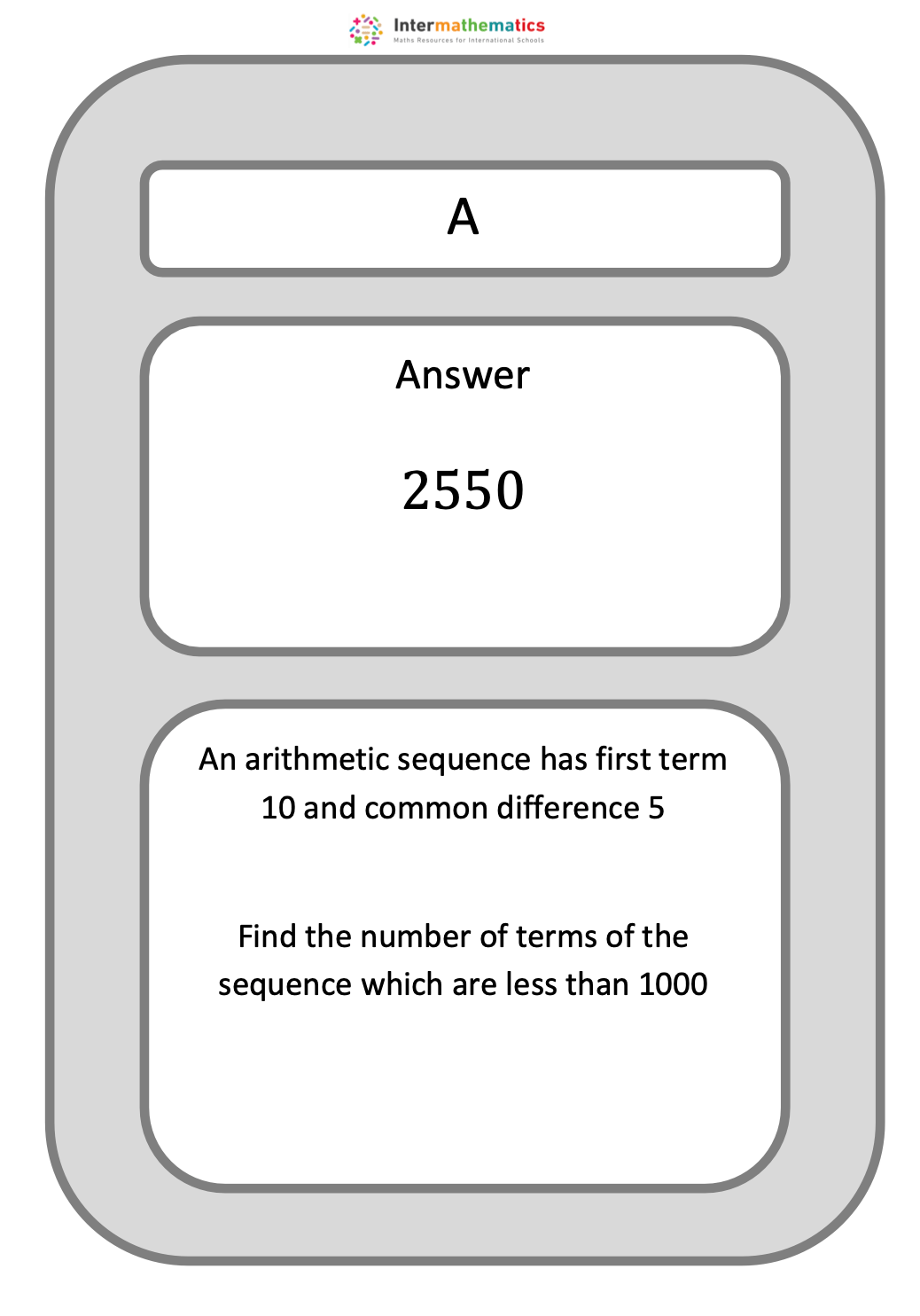Note:  This is an activity which can be done in pairs or as a whole class activity.  Students solve a mixture of arithmetic sequence problems.

Treasure Hunt:  Geometric sequences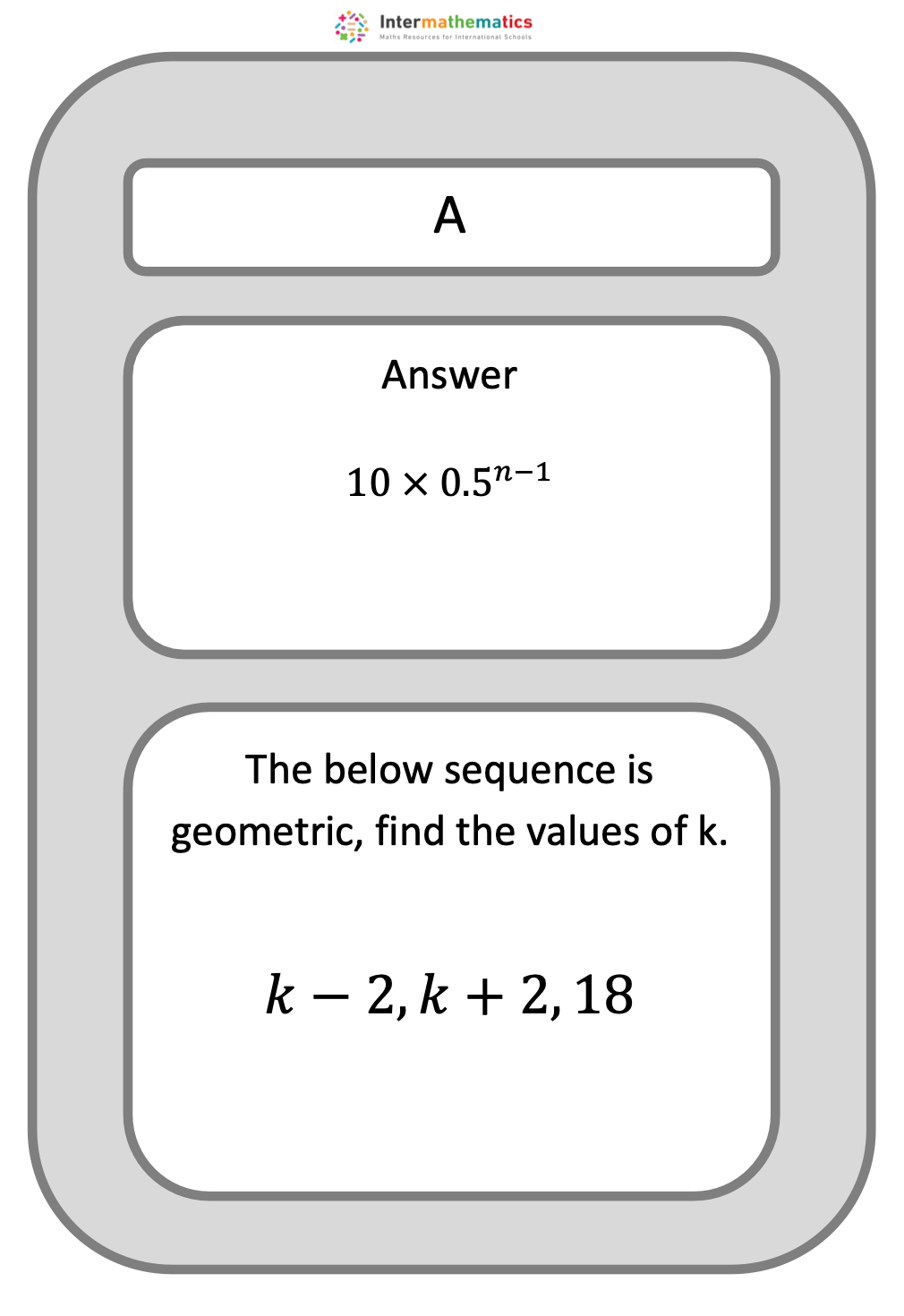Note:  This is an activity which can be done in pairs or as a whole class activity.  Students solve a mixture of Geometric sequence problems.

Treasure hunt compound interest 2022/09/24
Treasure hunt sequences geometric 2022/09/24
Treasure hunt sequences arithmetic 2022/11/04
Sequence puzzles (short) 2021/09/27
Mixed sequences HL 2021/08/16
Mixed sequences SL 2021/08/20